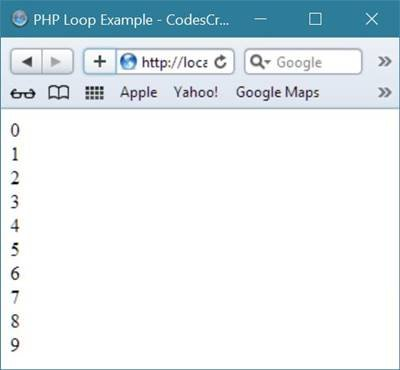# PHP Loops

Loops in PHP are used to executed the same block of code several number of times as specified.

## Types of Loop in PHP

There are following types of loop available in PHP:

You will learn all about the above loop types in detail devided in separate tutorial. For now, let's take a look at an example on looping technique in PHP.

## PHP Loop Example

Here is an example demonstrates how to use loop in a PHP program and how looping technique works.

```<!DOCTYPE html>
<html>
<title>PHP Loop Example - CodesCracker</title>
<body>
<?php
for(\$i=0; \$i<10; \$i++)
{
echo \$i;
echo "<br/>";
}
?>
</body>
</html>```

As you can see from the above loop example code in PHP, the variable \$i is initialized with 0 and checked whether it is less than 10 or not. As 0 is less than 10, then the condition becomes true and the block of code present inside the for loop will be executed, which is

```echo \$i;
echo "<br/>";```

This two line of code will print the value of variable \$i and then break the line using br tag.

Now after executing the code, again the program control goes to the updating part of the loop which is \$i++ here, after updating the value of variable \$i, again check whether the current value of \$i is less than 10 or not, if \$i will be less than 10 then again the program control goes inside the loop and execute the same code again which will again print the value of \$i and break the line. This flow of control continues until the condition evaluates to be false, here when the value of \$i becomes 10, then the condition evaluates to be false, as 10 is not less than 10. And the loop exits.

Here is the sample output produced by the above loop example code in PHP.You can write the same code using while loop as shown in the following program:

```<!DOCTYPE html>
<html>
<title>PHP Loop Example - CodesCracker</title>
<body>
<?php
\$i=0;
while(\$i<10)
{
echo \$i;
echo "<br/>";
\$i++;
}
?>
</body>
</html>```

The above PHP example code will produce the same output as the above one produced using the for loop.

Tools
Calculator